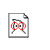# Neural Network Synthesis using Cellular Encoding and the Genetic Algorithm

Created by W.Langdon from gp-bibliography.bib Revision:1.5323

@PhdThesis{Gruau:1994:thesis,
• author = "F. Gruau",
• title = "Neural Network Synthesis using Cellular Encoding and the Genetic Algorithm",
• school = "Laboratoire de l'Informatique du Parallilisme, Ecole Normale Supirieure de Lyon",
• year = "1994",
• keywords = "genetic algorithms, genetic programming",
• URL = "ftp://ftp.ens-lyon.fr/pub/LIP/Rapports/PhD/PhD1994/PhD1994-01-E.ps.Z",
• URL = "ftp://ftp.ens-lyon.fr/pub/LIP/Rapports/PhD/PhD1994/PhD1994-01-F.ps.Z",
• size = "151 pages",
• abstract = "Artificial neural networks used to be considered only as a machine that learns using small modifications of internal parameters. Now this is changing. Such learning method do not allow to generate big neural networks for solving real world problems. This thesis defends the following three points:

(1) The key word to go out of that dead-end is {"}modularity{"}. (2) The tool that can generate modular neural networks is cellular encoding. (3) The optimization algorithm adapted to the search of cellular codes is the genetic algorithm.

The first point is now a common idea. A modular neural network means a neural network that is made of several sub-networks, arranged in a hierarchical way. For example, the same sub-network can be repeated. This thesis encompasses two parts.

The first part demonstrates the second point. Cellular encoding is presented as a machine language for neural networks, with a theoretical basis (it is a parallel graph grammar that checks a number of properties) and a compiler of high level language. The second part of the thesis shows the third point. Application of genetic algorithm to the synthesis of neural networks using cellular encoding is a new technology. This technology can solve problems that were still unsolved with neural networks. It can automatically and dynamically decompose a problem into a hierarchy of sub-problems, and generate a neural network solution to the problem. The structure of this network is a hierarchy of sub-networks that reflects the structure of the problem. The technology allows to experience new scientific domains like the interaction between learning and evolution, or the set up of learning algorithms that suit the GA.",

• notes = "

",

}

Genetic Programming entries for Frederic Gruau